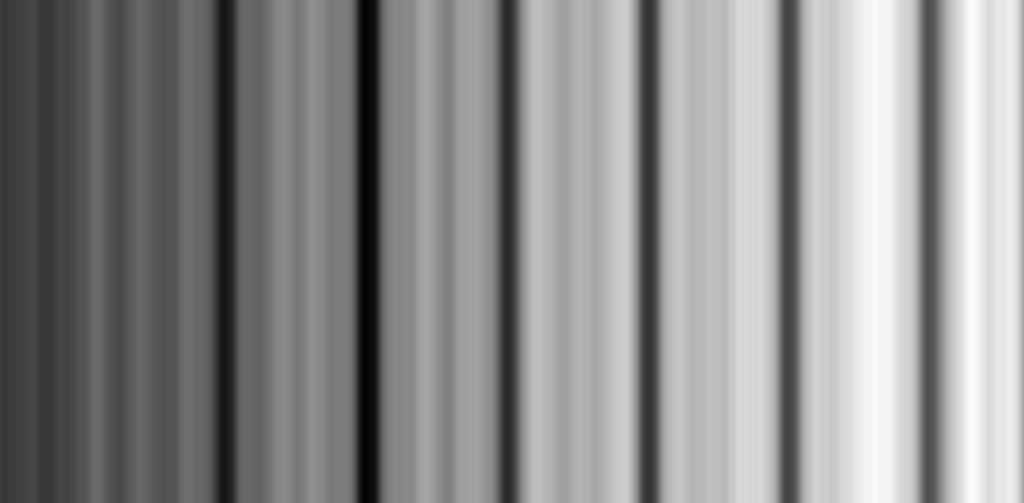## Monday, 20 January 2014

### Single nm linewidth measurement

My girlfriend Marijke Scotuzzi is a PhD candidate at the Delft university of technology.

Her main goal is to deposit small structures in the sub-10nm range.

She deposited some lines next to each other and she needs to calculate the width of those lines: the figure below shows an example of those lines. You may noticethat it is affected by an heavy high frequency noise.
Furthermore the lines can hardly be distinguished from the back spots which lies on the surface around them.

I've tried to help her out by writing a C++ program to enhance the original image.
Luckily I can make some assumpions on the input image: the lines are always vertical an they cross the top and the bottom limit edge of the image.

I've developed the following algorithm:
1. Apply a median blur to the image (optional) $Image[j][i] = medianBlur(Image[j][i])$
2. Compute a per-column weight $W[i] = \sum_{j=0}^J MedianImage[j][i]^2$
3. Apply a bilateral filter on vector W (optional) $W[i] = bilateralFilter(W[i])$
4. Compute the enhanced image $EnhancedImage[j][i] = Image[j][i]*\min((satO+W[i])*satM,255)$

Here below some results are shown for a couple of images:OutputMedian blurWeightsBilateral weights